# Finding Asymptotes Using Limits

Lesson Transcript
Instructor: Christopher Haines
The asymptotes of a function can be determined based on the limits of the given function. Learn about the different types of asymptotes and how to find the asymptotes of a given polynomial function. Updated: 12/14/2021

## What are Asymptotes?

To begin this topic, we first define all types of asymptotes.

#### Definition 1: Vertical Asymptote

A function f is said to have a vertical asymptote at x = a if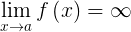or if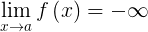These limits can be one-sided or two-sided.

#### Definition 2: Horizontal Asymptote

A function f is said to have a horizontal asymptote at y = b if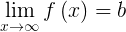or if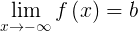#### Definition 3: Linear Asymptote

A function f is said to have a linear asymptote along the line y = ax + b if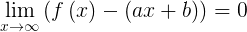or if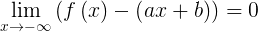A horizontal asymptote is a special case of a linear asymptote. This is the case of a = 0.

So, as we've seen with these three types of asymptotes, the concept of an asymptote (whether vertical, horizontal, or linear) is a function moving along a line in the limit as x approaches a finite number or positive or negative infinity. In the examples that follow, we're going to use the method of limits to locate asymptotes of various functions.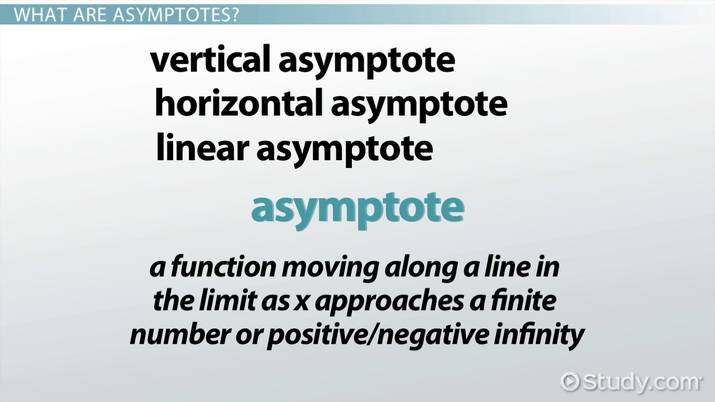An error occurred trying to load this video.

Try refreshing the page, or contact customer support.

Coming up next: Comparing Relative Magnitudes of Functions

### You're on a roll. Keep up the good work!

Replay
Your next lesson will play in 10 seconds
• 0:04 What Are Asymptotes?
• 1:31 Finding a Function's…
• 6:48 Lesson Summary
Save Save

Want to watch this again later?

Timeline
Autoplay
Autoplay
Speed Speed

## Finding a Function's Asymptotes

Here's our first example: Find all asymptotes for the function: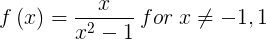You can sketch the function by using a graphing calculator.

Now here's our solution:

By dividing the numerator and denominator by x, we can evaluate the limit as x approaches infinity: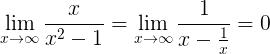Similarly,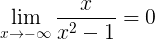In accordance with Definition 3, f has a horizontal asymptote at y = 0, and it has it in both directions towards positive and negative infinity.

Now note that by factoring the denominator, we can rewrite the function as: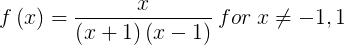With the function expressed this way, it becomes apparent that there are two suspects for vertical asymptotes. This is because x = -1 and x = 1 give a zero in the denominator. So we investigate these two points further and also be mindful of which side we are approaching each of the two points.

The one-sided limits are as follows. When x < -1, all three of the factors (numerator and denominator combined) are negative. So therefore: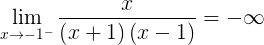When -1 < x < 0, two of the factors are negative, and one of the factors is positive, so the function is positive on this interval, and the right-sided limit at x = -1 is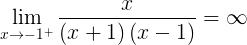Similarly for the other candidate,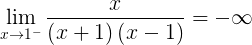and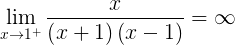The graph of the function is shown in Figure 1. The asymptotes in the plot are consistent with our calculations. However, sketching the plot in its entirety (without a graphing utility) is outside the scope of this lesson.

Figure 1: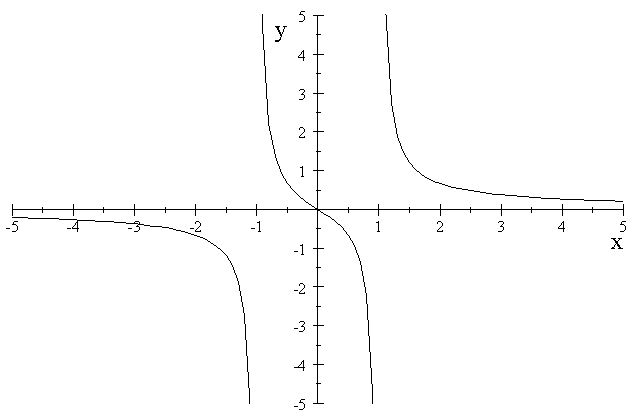Here is our second example: Find all asymptotes for the function: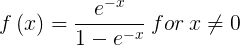Again, let's sketch the graph using a graphing calculator.

Now here's our solution:

Note that this function is really the same as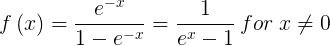We can obtain the latter expression upon multiplication on the numerator and denominator by exp(x). Clearly,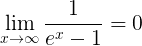and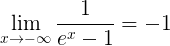So the function has two horizontal asymptotes: one for each direction of positive and negative infinity. They are y = 0 and y = -1.

Since the denominator is zero when x = 0, the only candidate for a vertical asymptote is x = 0. We will need to consider both one-sided limits as x approaches zero.

When x < 0, exp(x) < 1, so the left sided limit is then: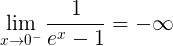And, similarly, when x > 0, exp(x) > 1. So the right sided limit is: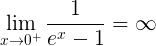To unlock this lesson you must be a Study.com Member.

### Register to view this lesson

Are you a student or a teacher?